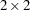Overview: FREQ Procedure

The FREQ procedure produces one-way to n-way frequency and contingency (crosstabulation) tables. For two-way tables, PROC FREQ computes tests and measures of association. For n-way tables, PROC FREQ provides stratified analysis by computing statistics across, as well as within, strata.

For one-way frequency tables, PROC FREQ computes goodness-of-fit tests for equal proportions or specified null proportions. For one-way tables, PROC FREQ also provides confidence limits and tests for binomial proportions, including tests for noninferiority and equivalence.

For contingency tables, PROC FREQ can compute various statistics to examine the relationships between two classification variables. For some pairs of variables, you might want to examine the existence or strength of any association between the variables. To determine if an association exists, chi-square tests are computed. To estimate the strength of an association, PROC FREQ computes measures of association that tend to be close to zero when there is no association and close to the maximum (or minimum) value when there is perfect association. The statistics for contingency tables include the following:

• chi-square tests and measures

• measures of association

• risks (binomial proportions) and risk differences fortables

• odds ratios and relative risks fortables

• tests for trend

• tests and measures of agreement

• Cochran-Mantel-Haenszel statistics

PROC FREQ computes asymptotic standard errors, confidence intervals, and tests for measures of association and measures of agreement. Exact p-values and confidence intervals are available for many test statistics and measures. PROC FREQ also performs analyses that adjust for any stratification variables by computing statistics across, as well as within, strata for n-way tables. These statistics include Cochran-Mantel-Haenszel statistics and measures of agreement.

In choosing measures of association to use in analyzing a two-way table, you should consider the study design (which indicates whether the row and column variables are dependent or independent), the measurement scale of the variables (nominal, ordinal, or interval), the type of association that each measure is designed to detect, and any assumptions required for valid interpretation of a measure. You should exercise care in selecting measures that are appropriate for your data.

Similar comments apply to the choice and interpretation of test statistics. For example, the Mantel-Haenszel chi-square statistic requires an ordinal scale for both variables and is designed to detect a linear association. The Pearson chi-square, on the other hand, is appropriate for all variables and can detect any kind of association, but it is less powerful for detecting a linear association because its power is dispersed over a greater number of degrees of freedom (except fortables).

For more information about selecting the appropriate statistical analyses, see Agresti (2007) or Stokes, Davis, and Koch (2000).

Several SAS procedures produce frequency counts; only PROC FREQ computes chi-square tests for one-way to n-way tables and measures of association and agreement for contingency tables. Other procedures to consider for counting include the TABULATE and UNIVARIATE procedures. When you want to produce contingency tables and tests of association for sample survey data, use PROC SURVEYFREQ. See Chapter 14, Introduction to Survey Sampling and Analysis Procedures, for more information. When you want to fit models to categorical data, use a procedure such as CATMOD, GENMOD, GLIMMIX, LOGISTIC, PROBIT, or SURVEYLOGISTIC. See Chapter 8, Introduction to Categorical Data Analysis Procedures, for more information.

PROC FREQ uses the Output Delivery System (ODS), a SAS subsystem that provides capabilities for displaying and controlling the output from SAS procedures. ODS enables you to convert any of the output from PROC FREQ into a SAS data set. See the section ODS Table Names for more information.

PROC FREQ uses ODS Graphics to create graphs as part of its output. For general information about ODS Graphics, see Chapter 21, Statistical Graphics Using ODS. For specific information about the statistical graphics available with the FREQ procedure, see the PLOTS= option in the TABLES statement and the section ODS Graphics.